# HBSE 8th Class Maths Solutions Chapter 6 Square and Square Roots Ex 6.3

Haryana State Board HBSE 8th Class Maths Solutions Chapter 6 Square and Square Roots Ex 6.3 Textbook Exercise Questions and Answers.

## Haryana Board 8th Class Maths Solutions Chapter 6 Square and Square Roots Exercise 6.3

Question 1.
What could be the possible ‘one’s’ digits of the square root of each of the following numbers ?
(i) 9801
(ii) 99856
(iii) 998001
(iv) 657666025
Solution:
(i) 98012 = 9801 × 9801
Possible one’s digits =1 [∵ 1 × 1 = 1]

(ii) 998562 = 99856 × 99856
Possible one’s digits =6 [∵ 6 × 6 = 36]

(iii) 9980012 = 998001 × 998001
∴ Possible one’s digits = 1

(iv) 6576660252 = 657666025 × 657666025
∴ Possible one’s placed digit = 5 [∵ 5 × 5 = 25]

Question 2.
Without doing any calculation, fin#the numbers which are surely not perfect squares.
(i) 153
(ii) 257
(iii) 408
(iv) 441
Solution:
(i) 153, (ii) 257 and (iii) 408 are surely not perfect squares.
[∵ The numbers end with 2, 3, 7, or 8 can never be a perfect square.]Question 3.
Find the square roots of 100 and 169 by the method of repeated subtraction.
Solution:
For $$\sqrt {100}$$
(i) 100 – 1 = 99
(ii) 99 – 3 = 96
(iii) 96 – 5 = 91
(iv) 91 – 7 = 84
(v) 84 – 9 = 75
(vi) 75 – 11 = 64
(vii) 64 – 13 = 51
(viii) 51 – 15 = 36
(ix) 36 – 17 = 19
(x) 19 – 19 = 0

For $$\sqrt {169}$$
(i) 169 – 1 = 168
(ii) 168 – 3 = 165
……………………….
………………………..
……………………….
………………………..
……………………….
……………………….
………………………
(xiii) 23 – 23 = 0
From 100 and 169 we have subtracted successive odd numbers starting from 1 and obtain 0 at 10th and 13th step.
∴ $$\sqrt {100}$$ = 10
and $$\sqrt {169}$$ = 13

Question 4.
Find the square roots of the follow¬ing numbers by the Prime Factorisation Method.
(i) 729
(ii) 400
(iii) 1764
(iv) 4096
(v) 7744
(vi) 9604
(vii) 5929
(viii) 9216
(ix) 529
(x) 8100.
Solution: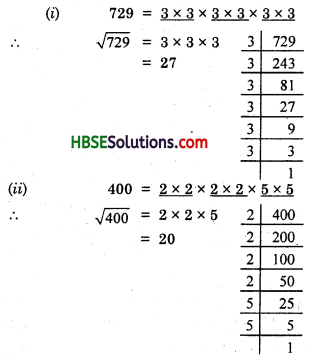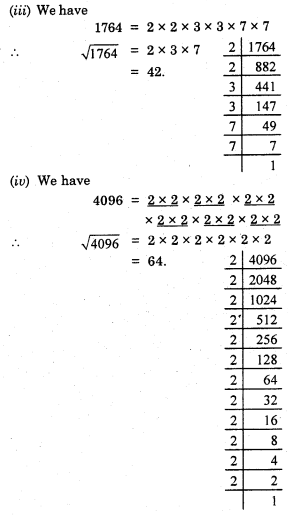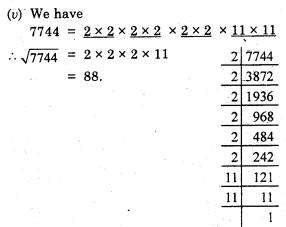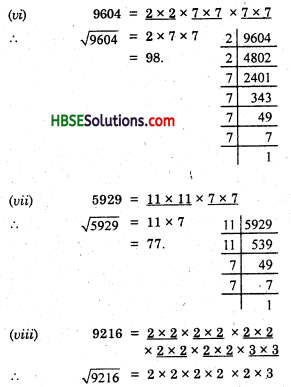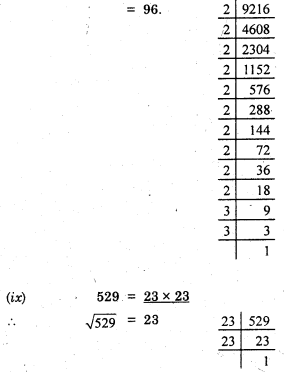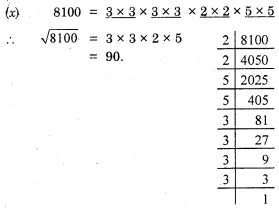Question 5.
For each of the following numbers, find the smallest whole number by which it should be multiplied so as to get a perfect square number. Also find the square root of the square number so obtained.
(i) 252
(ii) 180
(iii) 1008
(iv) 2028
(v) 1458
(vi) 768
Solution:
By prime factorisation, we get
252 = $$\underline{2 \times 2}$$ × $$\underline{3 \times 3}$$ × 7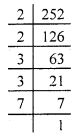We see that in prime factorisation of 252, there exists a number 7 which is unpaired.
Hence, we will have to multiply 252 by 7 to make a pair of 7.
∴ 252 × 7 = $$\underline{2 \times 2}$$ × $$\underline{3 \times 3}$$ × $$\underline{7 \times 7}$$
$$\sqrt {1764}$$ = 2 × 3 × 7
= 42.

(ii) By prime factorisation, we get
180 = $$\underline{2 \times 2}$$ × $$\underline{3 \times 3}$$ × 5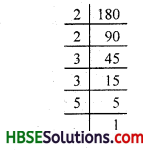The number 5 is unpaired.
∴ The smallest number by which 180 should be multiplied to make it a perfect square is 5.
Now,
180 × 5 = $$\underline{2 \times 2}$$ × $$\underline{3 \times 3}$$ × $$\underline{5 \times 5}$$
∴ 900 = $$\underline{2 \times 2}$$ × $$\underline{3 \times 3}$$ × $$\underline{5 \times 5}$$
∴ $$\sqrt {900}$$ = 2 × 3 × 5 = 30.

(iii) 1008 = $$\underline{2 \times 2}$$ × $$\underline{2 \times 2}$$ × $$\underline{3 \times 3}$$ × 7.
Here, 7 is unpaired
∴ We should multiply 1008 by to make it a perfect square.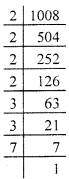Now,
1008 × 7 = $$\underline{2 \times 2}$$ × $$\underline{2 \times 2}$$ × $$\underline{3 \times 3}$$ × $$\underline{7 \times 7}$$
⇒ 1056 = 2 × 2 × 2 × 2 × 3 × 3 × 7 × 7
or $$\sqrt {1056}$$ = 2 × 2 × 3 × 7
= 84.

(iv) 2028 = $$\underline{2 \times 2}$$ × 3 × $$\underline{13 \times 13}$$
We see that the number 3 is unpaired.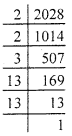∴ We should multiply 2028 by 3 to make it a perfect square.
Now,
2028 × 3 =$$\underline{2 \times 2}$$ × $$\underline{3 \times 3}$$ × $$\underline{13 \times 13}$$
Thus, 2084 = 2 × 2 × 3 × 3 × 13 × 3
∴ $$\sqrt {2084}$$ = 2 × 3 × 13 = 78

(v) 1458 = 2 × $$\underline{3 \times 3}$$ × $$\underline{3 \times 3}$$ × $$\underline{3 \times 3}$$.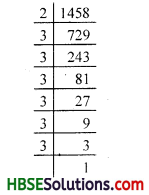Since a number 2 is unpaired.
So, smallest number by which 1458 should be multiplied is 2.
∴ Required number
= 1458 × 2
= 2916
Thus,
2916 = $$\underline{2 \times 2}$$ × $$\underline{3 \times 3}$$ × $$\underline{3 \times 3}$$ × $$\underline{3 \times 3}$$
$$\sqrt {2916}$$ = 2 × 3 × 3 × 3 = 54

(vi) By prime factorisation, we get
768 = $$\underline{2 \times 2}$$ × $$\underline{2 \times 2}$$ × $$\underline{2 \times 2}$$ × $$\underline{2 \times 2}$$ × $$\underline{3 \times 3}$$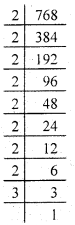Since a number 3 is unpaired.
∴ We should multiply 768 by 3 to make it a perfect square .
Now,
768 × 3 = $$\underline{2 \times 2}$$ × $$\underline{2 \times 2}$$ × $$\underline{2 \times 2}$$ × $$\underline{2 \times 2}$$ × $$\underline{3 \times 3}$$
⇒ $$\sqrt {2304}$$ = 2 × 2 × 2 × 2 × 3 = 48.Question 6.
For each of the following numbers, find the smallest whole number by which it should be divided so as to get a perfect square. Also find the square root of the square number so obtained.
(i) 252
(ii) 2925
(iii) 396
(iv) 2645
(v) 2800
(vi) 1620
Solution:
(i) We have,
252 = $$\underline{2 \times 2}$$ × $$\underline{3 \times 3}$$ × 7 (by prime factorisation)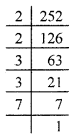If we divide 252 by 7 then
252 ÷ 7 = 36 = 2 × 2 × 3 × 3
which is a perfect square.
Thus, the required smallest number is 7
and $$\sqrt {36}$$ = 2 × 3 = 6

(ii) We have
2925 = $$\underline{5 \times 5}$$ × $$\underline{3 \times 3}$$ × 13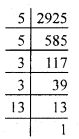Since a number 13 is unpaired.
We should multiply 768 by 3 to make it a perfect square .
∴ 2925 ÷ 13 = 225
= $$\underline{5 \times 5}$$ × $$\underline{3 \times 3}$$
which is a perfect square.
∴ The required smallest number is 13.
And $$\sqrt {225}$$ = $$\sqrt{5 \times 5 \times 3 \times 3}$$
= 5 × 3 = 15

(iii) By prime factorisation, we get
396 = $$\underline{2 \times 2}$$ × $$\underline{3 \times 3}$$ × 11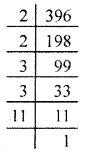If we divide 396 by 11, then
396 ÷ 11 = 36 = 2 × 2 × 3 × 3
which is a perfect square.
∴ The required smallest number is 11.
And, $$\sqrt {36}$$ = 2 × 3 = 6

(iv) 2645 = 5 × $$\underline{23 \times 23}$$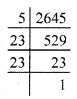Since a number 5 is a unpaired.
So, we should divide 2645 by 5.
2645 ÷ 5 = 529 = 23 × 23
which is a perfect square.
∴ The required smallest number = 5
and $$\sqrt {529}$$ = 23.

(v) By prime factorisation, we get
2800 = $$\underline{2 \times 2}$$ × $$\underline{2 \times 2}$$ × $$\underline{5 \times 5}$$ × 7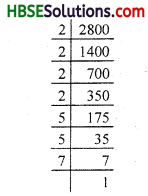If we divide 2800 by 7, then
2800 ÷ 7 = 400
= $$\underline{2 \times 2}$$ × $$\underline{2 \times 2}$$ × $$\underline{5 \times 5}$$
which is a perfect square.
Therefore, the required smallest number 7.
And, $$\sqrt {400}$$ = 2 × 2 × 5 = 20

(vi) By prime factorisation, we get
1620 = $$\underline{2 \times 2}$$ × $$\underline{3 \times 3}$$ × $$\underline{3 \times 3}$$ × 5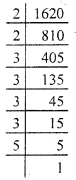Since a number 5 is unpaired.
∴ We should divide 1620 by 5.
1620 ÷ 5 = 324
= $$\underline{2 \times 2}$$ × $$\underline{3 \times 3}$$ × $$\underline{3 \times 3}$$
which is a perfect square.
Thus, $$\sqrt {324}$$ = 2 × 3 × 3 = 18.Question 7.
The students of Class VIII of a school donated Rs. 2401 in all, for Prime Minister’s National Relief Fund. Each student donated as many rupees as the number of students-in the class. Find the number of students in the class.
Solution:
Let the number of students in the class VIII be x.
Then, the rupees donated by each students will be Rs. x.
According to the given condition of the question :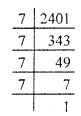x × x = 2401
⇒ x2 = 2401
⇒ x = $$\sqrt{2401}$$
= $$\sqrt{7 \times 7 \times 7 \times 7}$$
= 7 × 7
Hence, the number of students in the class is 49.

Question 8.
2025 plants are to be planted in a garden in such a way that each row contains as many plants as the number of rows. Find the number of rows and the number of plants in each row.
Solution:
Let the no. of plants contains in each row be x.
Then, the no. of rows will be also x.
∴ According to the given condition of the question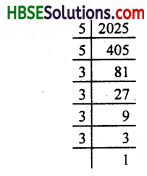Thus, x × x = 2025
⇒ x2 = 2025
⇒ x2 = 52 × 32 × 32
⇒ x2 = (5 × 3 × 3)2
⇒ x2 = 452
x = 45.
∴ The number of rows and the number of plants in each row is 45.Question 9.
Find the smallest square number that is divisible by each of the number 4, 9 and 10.
Solution:
This has to be done in three steps.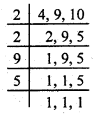Step 1. First of all find the LCM of the numbers 4,9 and 10.
LCM of 4, 9 and 10 = 180
Step 2. Then, find the prime factorisation of the LCM so obtained i.e., 180.
180 = $$\underline{2 \times 2}$$ × $$\underline{3 \times 3}$$ × 5
Step 3. Now, multiply the number 180 by 5 to make it a perfect square.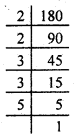⇒ 180 × 5 = $$\underline{2 \times 2}$$ × $$\underline{3 \times 3}$$ × $$\underline{5 \times 5}$$
⇒ 900 = $$\underline{2 \times 2}$$ × $$\underline{3 \times 3}$$ × $$\underline{5 \times 5}$$
Now, 900 is obviously a perfect square.
So, the required square number is 900.

Question 10.
Find the smallest square number that is divisible by each of the number 8, 15 and 20.
Solution:
Follow the same procedure as explained in the solution of Question (9)
LCM of 8, 15 and 20 = 120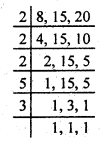Prime factorisation of 120 = $$\underline{2 \times 2}$$ × 2 × 3 × 5
We see that 2, 3 and 5 are not in pair.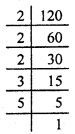Therefore, 120 should be multiplied by 2 × 3 × 5 i.e., 30 in order to get a perfect square.
Hence, the required square number is
120 × 30 = 3600.